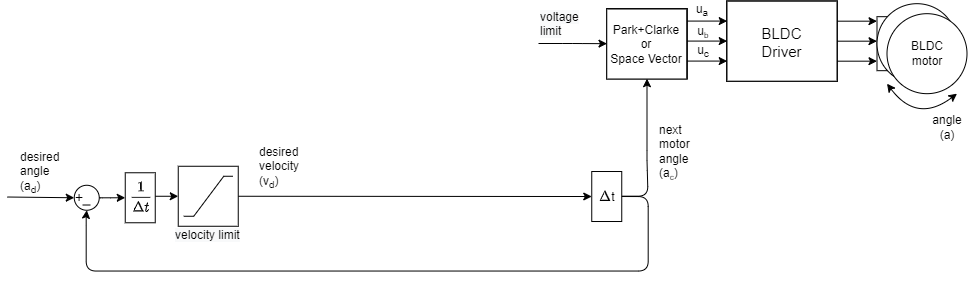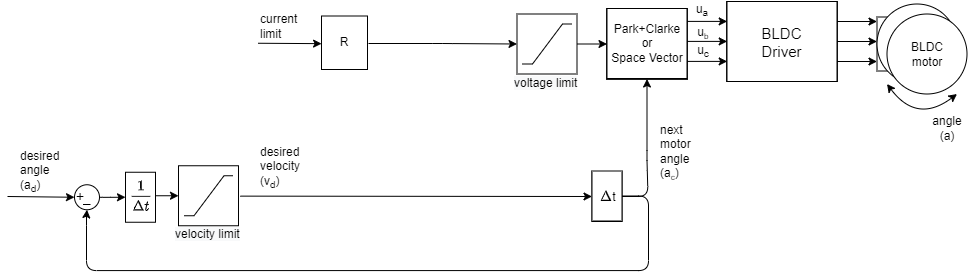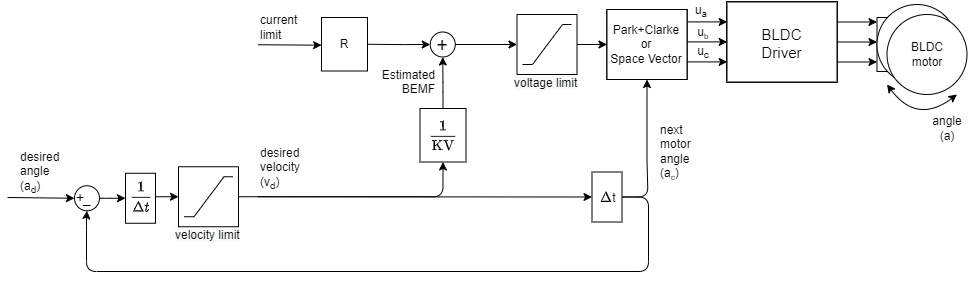# Position open-loop control

This control loop allows you to move your motor to the desired angle in real time without using the position sensor. This mode is enabled by:

// set position motion control open-loop
motor.controller = MotionControlType::angle_openloop;You can test this algorithm by running the examples in the motion_control/open_loop_motor_control/ folder.

This control algorithm is very simple. User sets the target angle it wants to achieve ad. The algorithm only subtracts the current angle ac and the desired angle ad to find the direction it needs to move and goes in that direction with the highest velocity possible motor.velocity_limit(max velocity). To set this velocity it uses the same algorithm as for velocity open-loop control. It integrates the velocity it in time to find out what is the angle it needs to set to the motor ac in order to achieve it. Then the maximal allowed voltage motor.voltage_limit is going to be applied in the direction of the ac using SinePWM or SpaceVector modulation.

// calculate the distance from the target
d_angle = target_angle - past_angle;
// constrain the distance with maximal allowable displacement
d_angle = constrain(d_angle, -velocity_limit*d_time, velocity_limit*d_time)
// calculate the next angle
next_angle = past_angle + d_angle;


## Configuration

// choose FOC modulation (optional)
motor.foc_modulation = FOCModulationType::SpaceVectorPWM;

//  maximal velocity of the position control
// default 20
motor.velocity_limit = 20;

// limiting voltage
motor.voltage_limit = 3;   // Volts
// or current  - if phase resistance provided
motor.current_limit = 0.5 // Amps



The angle open-loop control will (if not provided phase resistance) set the voltage to the motor equal to the motor.voltage_limit

voltage = voltage_limit; // Volts


This is very inefficient, as for different motors with different phase resistances the same voltage values can produce wildly different currents. For gimbal motor, you can run it in the open loop with the voltage limits of 5-10 Volts and it will reach the currents of 0.5-2 amps as it has the phase resistance from 5-15 Ohms. For drone motors, the voltage limits should stay very low, under 1 volt. Because they have phase resisatnce of 0.05 to 0.2 Ohms.

### Current limiting approaches

We suggest you to provide the motor class with the phase_resistance value and set the motor.current_limit instead of the voltage limit. This current might be surpassed but at least you will know an approximate current your motor is drawing. You can calculate the current the motor is going to be producing by checking the motor resistance phase_resistance and evaluating:

voltage = current_limit * phase_resistance; // Amps


The best way to use this control strategy would be to provide both phase resistance value and KV rating of your motor. The the library would be able to calculate he back-EMF voltage and much more precisely estimate the consumed current. And with the current and the back-EMF current the library can set much more appropriate voltage to the motor.

voltage = current_limit*phase_resistance + desired_velocity/KV; // Amps


### Velocity limit

The maximal velocity motor.velocity_limit value is going to determine how fast your motor goes in between positions. The higher the value the faster the transition. But since we are turning the motor in open-loop we will not be able to know if the motor can follow the velocity. So make sure to put the velocity_limit value that is achievable for your motor. Also beware that for higher velocities and more holding torque you will need to increase the motor.voltage_limit or motor.current_limit variable as well.

### Changing limits in real-time

Also, you can change the voltage limit motor.voltage_limit (motor.current_limit) and transition velocity motor.velocity_limit in real-time if you need this kind of behavior in your application.

## Position open-loop control example

Here is one basic example of the velocity open-loop control with the complete configuration. The program will set the target position of 0 RAD and maintain it, and the user can change the target position using serial terminal.

// Open loop motor control example
#include <SimpleFOC.h>

// BLDC motor & driver instance
BLDCMotor motor = BLDCMotor(11);
BLDCDriver3PWM driver = BLDCDriver3PWM(9, 5, 6, 8);

// instantiate the commander
Commander command = Commander(Serial);
void doTarget(char* cmd) { command.scalar(&motor.target, cmd); }
void doLimitVolt(char* cmd) { command.scalar(&motor.voltage_limit, cmd); }
void doLimitVelocity(char* cmd) { command.scalar(&motor.velocity_limit, cmd); }

void setup() {

// driver config
// power supply voltage [V]
driver.voltage_power_supply = 12;
driver.init();
// link the motor and the driver

// limiting motor movements
motor.voltage_limit = 3;   // [V]
motor.velocity_limit = 5; // [rad/s] cca 50rpm
// open loop control config
motor.controller = MotionControlType::angle_openloop;

// init motor hardware
motor.init();
motor.initFOC();

Serial.begin(115200);